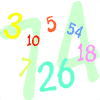### Math Rubric Generator

Directions: Fill in the information below.

STEP #1: SCHOOL, TITLE, AND TEACHER NAME

Enter the appropriate information in the correct box below. Please note that if the title is left blank, nothing will appear in the output.

 School Name:
 Title of Rubric:
 Teacher Name:

STEP #2: PICK A PICTURE

Choose one picture from the pictures below by:Monkey AroundGoing UpSuppliesTeacherBrainnyLast Minute

Once you have completed the form above, click the "GENERATE-MATH RUBRIC" button below to create your rubric.

## How to Use a Math Rubric

With numerous steps involved in equations and word problems, it's often possible to skip the actual critical thinking part of the solution and jump straight to grading on the answer's basis. However, math rubrics help you keep the steps in mind while making the grading comprehensive and effortless. Keep reading below to find out how a math rubric for solving equations and math word problems can aid students and teachers alike.

Rubric for Solving Math Word Problems

A math rubric will aid students in remembering steps and knowing forehand where they can lose scores. To develop a rubric for solving math word problems, here are the criteria you can include:

Comprehending the Problem

The initial and the most crucial step of solving a math problem is comprehending the problem entirely. Students can do so by using the given data and paying attention to the terminologies used in the questions.

They often indicate what steps are required to solve the problem. If a student is successful in this step, it would be evident with their way of gathering and noting the given information.

Planning a Solution

Before diving right into the solution, students must plan out one to navigate through the process of solving a word problem step by step.

This criterion ensures students do not skip a step that can often provide a faulty base for other steps and result in wrong answers and wasted effort. Teachers must be vigilant during this step and encourage students to plan the solution and then execute it.

Solving the Problem

This step is crucial as all the previous steps of the process lead to this part, whereas the final answer to the word problem relies upon this step. Students must use the given data and their prior knowledge to execute the previous step's planning.

Allow students to independently write down the solution step-by-step and compute the answer on its basis.

It doesn't have to be a eureka moment, and it's likely not to be, as students will find the answer based on the previous steps. Students will find it easy to calculate their answers by using a solid foundation of the earlier steps as support.

Grading students with a rubric makes the result a part of the process instead of the goal and helps students focus on more critical objectives, i. e. learning critical thinking and problem-solving.

Why Should You Use a Math Rubric?

Math word problems and equations can be tough to grade, with numerous steps playing an essential part in computing the actual solution. That's where rubrics play an integral role in grading, as it allows you to keep track of each step and its weightage in finding the solution.

It's often possible for students to skip the steps involved and only write the answer, eliminating a significant part of solving math problems: planning the solution. However, with the help of rubrics, students follow the order of steps. Using a math rubric also makes grading easier for the teacher.

Wrapping Up

Using a math rubric for solving equations is beneficial for students and allows you to grade students' answers efficiently. Similarly, having a preset rubric for solving math word problems can also make the process fast.

You can include the above-discussed criteria in your math rubric to make learning fun for your students and grading effortless for you.